• 线性回归模型建模步骤一元线性回归、多元线性回归）
• 来自烟水暖的学习笔记回归分析(Regression analysis)回归...回归分析的分类：1） 按自变量的个数，可以分为一元回归，多元回归2）按变量相关性的形状（回归线）是否为直线型，可分为线性回归，非线性回归。下面，...
来自烟水暖的学习笔记回归分析(Regression analysis)回归分析(Regression analysis)，是研究因变量与自变量之间相关性的一种数学方法，并将相关性量化,即得到回归方程。我们可以通过回归方程（回归预测模型）来预测因变量的变化。回归分析的分类：1） 按自变量的个数，可以分为一元回归，多元回归2）按变量相关性的形状（回归线）是否为直线型，可分为线性回归，非线性回归。下面，我们将通过python工具来理解最简单的回归分析，即一元线性回归，并了解回归分析的步骤。想要更多了解回归分析包含的内容，可参考下面内容烟水暖：详细解读Excel回归分析：价格与需求的相关性​zhuanlan.zhihu.com一元线性回归顾名思义，就是只包含一个自变量，且与因变量的关系是呈线性的回归分析，其回归方程（回归模型）可用下面的方程表示：y=bx+a （其中:y为因变量，x为自变量，b为回归系数或斜率，a为截距）简单实例我们将通过分析产品A的单价与销量之间的关系，来预测当产品A的定价为x，市场的需求量y将会是多少，这样我们就能知道该向供应商订购多少产品A。数据如下：确定变量，导入数据2. 探索性数据分析，绘制散点图，观察变量之间关系及趋势变化，确定回归类型#绘制散点图，观察Price,demand的相关性由图可见，Price与Demand具有较强的负相关性，即Price增加，Demand反而减少。我们也可通过下面函数观察Price与Demand的相关系数（相关系数R是描述变量之间的相关性，范围在[-1,1]之间，R>0，则为正相关，R<0，则为负相关；R的绝对值越接近于1，相关性越强，反之，相关性越弱）3. 建立回归模型，并进行模型训练在统计学中，一般我们是通过“最小二乘法”直线拟合来执行线性回归分析。4. 检验模型的拟合程度查看模型的判断系数，判断其拟合度。判断系数为0.8679788013877346，可见模型的拟合程度还是较高的。下面将拟合回归线放入散点图，直观的感受模型的拟合程度:最后查看回归方程的内容:模型包含的内容：参数：fit_intercept： 默认为true,是否计算截距normalize： 是否将数据归一化copy_X：是否复制x的值，默认是复制，否则覆盖原来x的值n_jobs：计算模型使用的作业数，也叫核数属性：coef_:斜率intercept_:截距方法：fit() 拟合线性模型get_params() 获取估计量的参数,即返回上面四个参数的内容predict() 使用模型预测结果score()  返回判定系数 ^2,判定系数描述的是回归方程的拟合优度，值越接近于1,拟合度越高set_params()设置估计量的参数的内容这里，我们只需要拿到下面内容，即可知道回归模型的内容。即Price与Demand呈负相关性，且可用 y=-0.895x+10.128来描述其关系。接下来，我们便可用这个模型来预测需求。5. 使用回归模型预测通过模型的预测方法，我们可预测当产品A的价格为8，或8.2，对应的需求为2.96，2.78.总结通过上面的学习，我们可用了解到最简单的线性回归分析，理解其如何帮我们来分析Price和Demand之间的关系，并由此预测Demand。
展开全文• 确定了一元线性方程中的n，就可以作为degree传入PolynomialFeatures类； 预测的时候不能直接使用转换前的因变量作为参数传入模型，需要fit_transform转换才能进行预测 1 from sklearn.linear_model ...
1、使用scatter_matrix判断个特征的数据分布及其关系散步矩阵(scatter_matrix)Pandas中散步矩阵的函数原理1 def scatter_matrix(frame, alpha=0.5, figsize=None, ax=None, diagonal='hist', marker='.', density_kwds=None,hist_kwds=None, range_padding=0.05, **kwds)参数如下：frame:(DataFrame)，DataFrame对象alpha:(float,可选)，图像透明度，一般取(0,1]figsize:((float,float),可选)，以英寸单位的图像大小，一般以元组(width,height)形式设置ax:(Matplotlib axis object,可选)，一般取Nonediagonal:({"hist","kde"})，必须只能从这两个中选一个，"hist"表示直方图(Histogram plot)，“kde”表示核密度估计(Kernel Density Estimation);该参数是scatter_matrix函数的关键参数marker：（str，可选），Matplotlib可用的标记类型，如：‘，’，‘.’，‘o’density_kwds：（other plotting keyword argumentss，可选），与kde相关的字典参数hist_kwds：(other plotting keyword arguments，可选)，与hist相关的字典参数range_padding：（float，可选），图像在x轴，y轴原点附近的留白(padding)，该值越大，留白距离越大，图像远离坐标原点kwds:(other plotting keyword argumentss，可选)与scatter_matrix函数本身相关的字典参数kde值diagonal参数取’kde’值时，表示散布矩阵的对角线上的图形为数据集各特征的核密度估计(Kernel Density Estimation，KDE)。核密度估计是在概率论中用来估计未知的密度函数，属于非参数检验方法之一。简单来说，核密度估计就是在当前数据集(连续型数据样本)已知的情况下，通过计算来获取该样本分布的概率密度函数；在计算获取时需要用到核函数，如Gaussian Kernel Density、Exponential Kernel Density、Cosine Kernel Density等，不同核函数可以得到样本整体趋势和密度分布规律性基本一致的结果；影响该结果的还包括带宽（bandwidth），带宽值过大或过小都会影响估计结果；---- 来自 Felix_YU_Q 的CSDN 博客 ，全文地址请点击：https://blog.csdn.net/hurry0808/article/details/78573585?utm_source=copy步骤：1、导入模块：pandas、matplotlib2、读取数据3、为项目设置matplotlib参数设置参数有两种方法：调用命令matplotlib.rc()；通过传入关键字元组，修改参数使用参数字典：matplotlib.rcparams；为了能让matplotlib能正常显示中文和能正常显示符号，需调用以下语句matplotlib.rcParams['font.sans-serif']=['SimHei']   #用来正常显示正文matplotlib.rcParams['axes.unicode_minus']=False    #用来正常显示负号4、使用scatter_matrix画图1 importpandas2 importmatplotlib34 data=pandas.read_csv(5             "C:\\Users\\Jw\\Desktop\\python_work\\Python数据挖掘实战课程课件\\4.3\\data.csv")67 x=data[["等级"]]8 y=data[["资源"]]910 font={11       "family":"SimHei"}1213 matplotlib.rc('font', **font);14 matplotlib.rcParams["axes.unicode_minus"]=False1516 from pandas.tools.plotting importscatter_matrix1718 scatter_matrix(19         data[["等级", "资源"]],20         alpha=0.8,figsize=(10,10),diagonal="kde")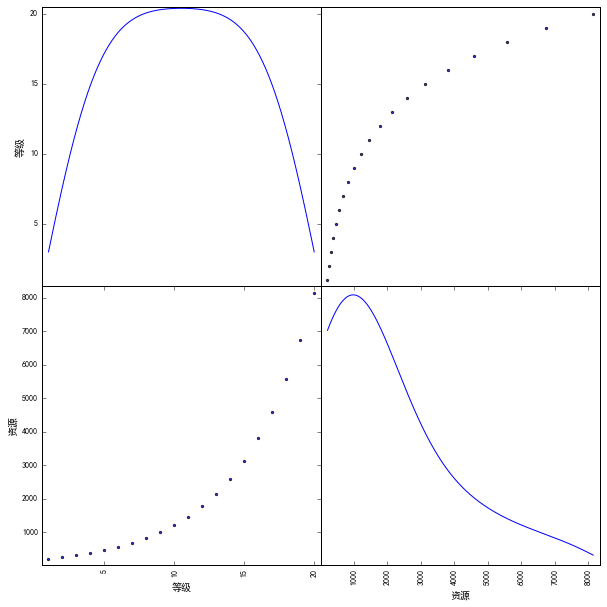2、根据得到的图形与已经的模型对比scatter_matrix得到的kde图符合一元二次方程，与一元二次方程进行对比1 importnumpy;2 x_ = numpy.arange(-10, 10, 0.01);3 y_ = x_**245 from matplotlib importpyplot as plt;67 plt.figure();8 plt.title('等级与资源')9 plt.xlabel('等级')10 plt.ylabel('资源')11 plt.grid(True)12 plt.plot(x_, y_, 'k.')13 plt.show()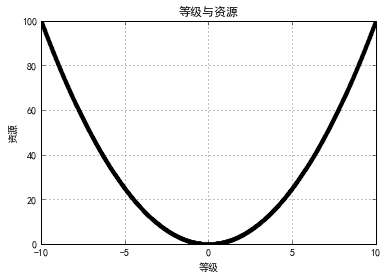与scatter_matrix得到的相符合，degree设置为23、使用PolynomialFeaturess来构建特征它是使用多项式的方法来进行的，如果有a，b两个特征，那么他的2次多项式为(1，a，b，a^2，b^2)PolynomialFeatures有三个参数：degree：空值多项式的度interaction_only:默认为False，如果指定为True，那么就不会有特征自己和自己结合的项，上面的二次项中没有a^2，b^2include_bias:默认为True，如果为True的话，就会有上面的1 那一项解决方法:把一元非线性方程转化为多元一次方程；确定了一元非线性方程中的n，就可以作为degree传入PolynomialFeatures类；预测的时候不能直接使用转换前的因变量作为参数传入模型，需要fit_transform转换才能进行预测1 from sklearn.linear_model importLinearRegression2 from sklearn.preprocessing importPolynomialFeatures345 pf=PolynomialFeatures(degree=2)6 x_2_fit=pf.fit_transform(x)  #一元二次的方程就转化为二元一次的方程78 lrModel=LinearRegression()9 lrModel.fit(x_2_fit,y)1011 lrModel.score(x_2_fit,y)    #模型拟合程度1213 x_2_predict=pf.fit_transform([,])14 lrModel.predict([,])小结：一元n次方程，转换为多元线性方程：pf=sklearn.preprocessing.PolynomialFeatures(degree=2)转换方法：x_2_fit=pf.fit_trasform(x)预测：LinearRegression().predict(x_2_fit)
展开全文• 模型：一元线性回归模型 回归分析：建立方程模拟两个或者多个变量之间是如何相互关联，被预测的变量称为因变量（结果），用来进行预测的变量称为 自变量（输入参数），当输入参数只有一个（输出1个）时，称为一元...
模型：一元线性回归模型

回归分析：建立方程模拟两个或者多个变量之间是如何相互关联，被预测的变量称为因变量（结果），用来进行预测的变量称为

自变量（输入参数），当输入参数只有一个（输出1个）时，称为一元回归，反之当输入有多个（输出1个），称为多元回归；

一元线性回归模型如下所示：（我们只需确定此方程的两个参数即可）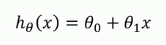第一个参数为截距，第二个参数为斜率（我们只需根据大量的数据集通过训练求解即可^_^）

为了求解上述参数（或许求解的参数更多）我们在这里引入代价函数（cost function）,在这里以一元线性回归模型为例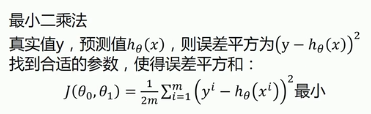上述式子不难理解，为真实值与预测值之间的差值的平方（当然也可以取绝对值，但为便于后续数值操作取其平方），最后

取所有训练集个数的平均值。而我们就是在取某个(些)参数时，使得上述函数取得最小值（误差最小！）结合下图直观理解。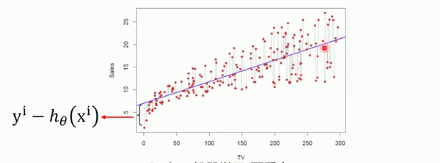上述由一些参数构成的函数称为代价函数，我们的目标就是求解对应的参数使得代价函数达到最小值，最后确定模型；梯度下降法（确定所要求解的参数，在神经网络模型中也会有所应用）： 其具体步骤如下图所示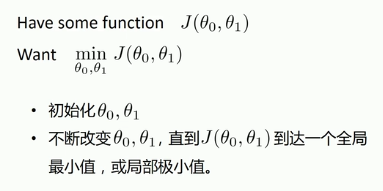使用此方法最重要的就是确定代价函数，且此函数可以收敛到最小值（凸函数），对于初始化操作，一般情况下赋值为0即可

所谓的梯度优化，就是不断的更改参数值，使之最后到达一个全局（局部）最小值，参数更新过程如下；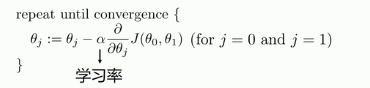一般情况下的参数求解，对每个参数求偏导数；

这一个参数更新要求是同步更新，即最后在对参数进行更新，这里的α为学习率，通常取值为0.01，0.001，0.03，0.003等

学习率不易过高也不能过低，当过高是会导致永远到达不了收敛点（发散），当过低时会导致收敛过慢，影响收敛速度；

在这里的一元线性回归模型我们确定的参数只有两个，其参数求解方式如下图所示：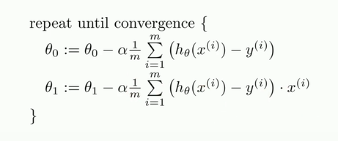实战 （fight）

在这里我们以一个生产成本对月产量的影响为例，确定两者之间的线性关系（当然未必一定是线性）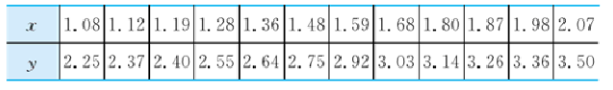下图红色部分是通过梯度下降求解出的拟合直线，蓝色为通过python自带的库sklearn拟合出的直线（两者大体相同）

import numpy as np  #导入numpy
import matplotlib.pyplot as plt #导入图像绘制库
from sklearn.linear_model import LinearRegression  #线性回归库

上述为我们常用的三个库文件的导入方式，numpy（矩阵运算），pyplot（图像绘制），sklearn

#训练集，以及相关参数
dataX=[]# X数据集
dataY=[]# Y数据集
with open('DataSet') as f:
for line in f:
line=line.strip('\n').split(' ')
dataX.append(float(line))
dataY.append(float(line))

k=0 # 斜率
b=0 # 截距  初始化参数
learnrate= 0.03 # 学习率
step = 50 # 学习次数

#梯度下降法求解参数
for i in range(step):
temp1=0
temp0=0
for j in range(len(dataX)):
temp0=temp0+(k*dataX[j]+b-dataY[j])/len(dataX)
temp1=temp1+(k*dataX[j]+b-dataY[j])*dataX[j]/len(dataX)
k=k-learnrate*temp1
b=b-learnrate*temp0 #求解参数

#通过sklearn库训练数据
model=LinearRegression()
model.fit(np.array(dataX).reshape(-1,1),np.array(dataY).reshape(-1,1))
plt.plot(dataX,model.predict(np.array(dataX).reshape(-1,1)),'b')

#绘制散点以及拟合直线图像
plt.xlabel('X')
plt.ylabel('Y')# 设置横纵坐标
plt.scatter(dataX,dataY)# 绘制散列表
plt.plot(dataX,k*np.array(dataX)+b,'r')# 绘制拟合直线
plt.show()


这里的scatter传入的参数为X和Y坐标列表，要求X和Y列表内元素个数相同，plot用于绘制曲线第一个参数为X坐标，第二

个参数为函数方程（Y坐标），第三个参数为曲线的颜色。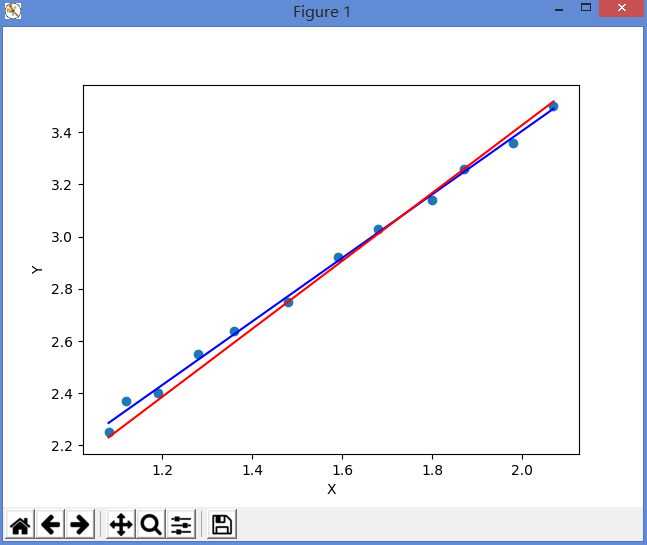拟合出的一元线性回归方程为：y=1.274974x+0.879003；

展开全文机器学习
• **TensorFlow 实现一元线性回归模型一元线性回归即是将N个随机样本点拟合到一条直线上：样本点： x y 3.3 1.7 4.4 2.7 5.5 2.1 6.71 3.2 6.93 1.69 4.168 1.573 9.779 3.366 6.182 2.596 … … ...
**

TensorFlow 实现一元线性回归模型

一元线性回归即是将N个随机样本点拟合到一条直线上：

样本点：

x
y
3.3
1.7
4.4
2.7
5.5
2.1
6.71
3.2
6.93
1.69
4.168
1.573
9.779
3.366
6.182
2.596
…
…
样本点可表示为：

(X,Y)N=[(x1,y1),(x2,y2)...(xn,yn)]<!--//--><![CDATA[//><!--
(X,Y)_N= [ (x_1,y_1),(x_2,y_2)...(x_n,y_n)  ]
//--><!]]>我需要拟合出的直线方程可以表示为：

y^=f(x)=Wx+b<!--//--><![CDATA[//><!--
\hat y = f(x) =Wx +b
//--><!]]>可以通过最小二乘法来求得拟合直线的值，拟合过程就是使得MSEloss的最小值。拟合过程就转化成求以下函数的极值问题。 MSEloss=minw,b12N∑n=1N(y^−yn)2<!--//--><![CDATA[//><!--
MSEloss = min_{w,b} \frac{1}{2N} \sum_{n=1}^N(\hat y -y_n)^2
//--><!]]>W就可表示为： W=(XTX)−1Y<!--//--><![CDATA[//><!--
W = {(X^TX)}^{-1}Y
//--><!]]>上式需要用所有样本来训练，训练过程也可采用另一种方式，使用随机梯度下降法求得极值，则不用一次入所有样本用于训练，步骤如下：
step0： 给定W ,b初始值，W0,b0<!--//--><![CDATA[//><!--
W_0,b_0
//--><!]]>,
step1： 随机选取一个样本 (xn,yn)<!--//--><![CDATA[//><!--
(x_n,y_n)
//--><!]]>，
step2： 将样本和Wt−1,bt−1<!--//--><![CDATA[//><!--
W_{t-1},b_{t-1}
//--><!]]>代入 y^n=ft−1(xn)=Wt−1xn+bt−1<!--//--><![CDATA[//><!--
\hat y_n = f_{t-1} (x_n)= W_{t-1}x_n+b_{t-1}
//--><!]]>确定y^n<!--//--><![CDATA[//><!--
\hat y_n
//--><!]]>,
step3： 求得Wt=Wt−1+α(y^n−yn)xn<!--//--><![CDATA[//><!--
W_t = W_{t-1} + \alpha(\hat y_n - y_n)x_n
//--><!]]>,
step4： 求得bt=yn−Wtxn<!--//--><![CDATA[//><!--
b_t= y_n - W_tx_n
//--><!]]>,
循环迭代1-4步，使得MSEloss的值小于某个值退出循环。

TensorFlow程序设计：

inference： 先确定线性拟合直线表达式
loss： 制定损失判定标准
train： 将损失函数代入梯度下降节点，n次迭代，获得W,b的权值
evaluate：将权值作用于测试点，计算损失。损失越小，模型越精确。

代码

import os
import tensorflow as tf
import numpy as np
import matplotlib.pyplot as plt
#os.environ['TF_CPP_MIN_LOG_LEVEL'] = '2'

train_X = np.asarray([3.3,4.4,5.5,6.71,6.93,4.168,9.779,6.182,7.59,2.167,
7.042,10.791,5.313,7.997,5.654,9.27,3.1])
train_Y = np.asarray([1.7,2.76,2.09,3.19,1.694,1.573,3.366,2.596,2.53,1.221,
2.827,3.465,1.65,2.904,2.42,2.94,1.3])

plt.plot(train_X,train_Y,'ro',label='train points')
plt.legend()
plt.show()

with tf.Graph().as_default():
#input
with tf.name_scope('Input'):
X = tf.placeholder(tf.float32 , name="X")
Y_true = tf.placeholder(tf.float32 , name="Y_true" )

with tf.name_scope('Inference'):
#模型参数变量
W = tf.Variable(tf.zeros(),name="Weight")
b = tf.Variable(tf.zeros(),name="Bias")
#inference ：Y= WX + b

with tf.name_scope('Loss'):
#loss :
Trainloss = tf.reduce_mean(tf.pow(Y_true - Y_pre,2))/2

with tf.name_scope('Train'):
#train:
TrainOp = optimizer.minimize(Trainloss)

with tf.name_scope('Evaluate'):
#evaluate:
Evaloss = tf.reduce_mean(tf.pow(Y_true - Y_pre,2))/2

InitOp = tf.global_variables_initializer()

writer = tf.summary.FileWriter(logdir='logs_1',graph=tf.get_default_graph())
writer.close()

print("start session  run graph, train start")

with tf.Session() as sess:
sess.run(InitOp)
for step in range(1000):
#for tx,ty in zip(train_X,train_Y):
# trainOp,trainloss,t_w,t_b = sess.run([TrainOp,Trainloss,W,b],feed_dict={X:tx,Y_true:ty})
trainOp,trainloss,t_w,t_b = sess.run([TrainOp,Trainloss,W,b],feed_dict={X:train_X,Y_true:train_Y})
if((step + 1)%5 == 0):
print("step:", (step+1),"train loss:","{:.9f}".format(trainloss),"W=",t_w,"b=",t_b)

print("train end")
W,b = sess.run([W,b])
print("para mode: W=",W," b=",b)

plt.plot(train_X,train_Y,'ro',label='train points')
plt.plot(train_X, W * train_X + b,label="Fitted Line")
plt.legend()
plt.show()

展开全文TensorFlow
• %stats是用于检验回归模型的统计量，有三个量， %第一个是决定系数R的平方，第二个是F统计量值，第三个是与F统计量值对应的概率P Z=b(1)+b(2)*X;%回归方程 plot(X,Y,'rp',X,Z,'b'); title('原始数据散点图与回归...
• R语言解读一元线性回归模型 R的极客理想系列文章，涵盖了R的思想，使用，工具，创新等的一系列要点，以我个人的学习和体验去诠释R的强大。 R语言作为统计学一门语言，一直在小众领域闪耀着光芒。直到大数据的...
• 什么是线性回归呢？ 线性回归是分析一个变量与另一个变量的关系的方法。 线性回归的意思就是指x与y之间的关系是线性的。 y = wx+b 求解的步骤： 1. 确定模型。 这里选择y = ...
• 一、线性回归模型介绍 简单来说：线性回归就是利用一曲线段对一些连续的数据进行拟合，进而可以用这条曲线预测新的输出值。数学模型如下： 其中：w称为权重，b称为偏置，利用现有的数据训练出理想的w和b的值，...TensorFlow python 深度学习 人工智能
• 在统计学中，线性回归（linear regression）是一种回归分析方法，它利用基于最小二乘函数的回归方程对一个或多个自变量和因变量之间的关系进行建模。 通过这个例子能够更加深入的理解TensorFlow的概念和使用方法。 ...TensorFlow 深度学习
• 一元线性回归分析是处理两个变量之间关系的最简单模型，它所研究的对象是两个变量之间的线性相关关系。通过对这个模型的讨论，我们不仅可以掌握有关一元线性回归的知识，而且可以从中了解回归分析方法的基本思想、...
• Excel一元线性回归示例 1 声明 本文的数据来自网络，部分代码也有所参照，这里做了注释和延伸，旨在技术交流，如有冒犯之处请联系博主及时处理。 2 一元线性回归简介 回归分析只涉及到两个变量的，称一元回归...
• 一元线性回归分析（SPSS版） 原创 Gently spss学习乐园 2019-11-25 一元线性回归模型回归分析（regression analysis ）是研究一个变量如何随另一个或一些变量变化的方法。例如，学习成绩会受努力的时间，方法，个人...
• 第11章 一元线性回归 11.1 变量间关系的度量 变量之间的关系可分为两种类型，即函数关系和相关关系。其中，函数关系是一一确定的关系，给定一个自变量x，因变量y依确定的关系取相应的值；变量之间存在的不确定性的...数理统计 数据分析 机器学习基础
• 回归（Regression） ”回归到中等“ 房价预测： 回归分析（regression analysis）用来建立方程模拟两个或者多个变量之间如何关联 被预测的变量叫做：因...一元线性回归包含一个自变量和一个因变量 以上两个变...机器学习
• 用Python外部扩展模块NumPy中数组的操作，并用NumPy、sklearn模块进行一元线性回归分析。 配置：Window 7旗舰版+64位操作系统+Anaconda3 Spyder（64-bit） 这篇博文是博主的实验报告，之所以以图片形式上传就是避免...python
• 损失函数 L1损失：基于模型预测的值与标签的实际值之差的绝对值 平方误差（L2误差）：均方误差（MSE）指每个样本的...步骤 准备数据 构建模型 训练模型 进行预测 生成人工数据集 #在jupyter中使用matplotlib显示图...机器学习 TensorFlow
• 最简单最基础的即是一元线性回归模型。通过一元线性回归可以衍生出了许多模型，所以学好一元线性回归是非常有必要的，是目前所有回归模型的基础。即使跳出统计、计量的领域，到了数据挖掘、人工智能的领域，线性回归...
• 实验二 一元线性回归模型的估计、检验、预测和应用-学生实验报告 (12页) 本资源提供全文预览，点击全文预览即可全文预览,如果喜欢文档就下载吧，查找使用更方便哦！17.90 积分飞矢告牌葬秸笆麦蜘楔揭许排漓莹遏避泽...
• ## SPSS一元线性回归

千次阅读 2020-02-13 11:22:12
回归分析是数据分析中最基础也是最重要的分析工具，绝大多数的数据分析问题，都可以使用回归的思想来解决。 回归分析的任务就是：通过研究自变量X和因变量Y的相关关系，尝试去解释Y的形成机制，进而达到通过X去预测...数据分析 大数据
• 一元线性回归分析是在排除其他影响因素，分析某一个因素（自变量：X）是如何影响另外一个事物（因变量：Y）的过程，所进行的分析是比较理想化的。对于一元线性回归来说，可以看成Y的值是随着X的值变化，每一个实际的...matlab
• 基于EXCEL的一元线性回归案例：广告与销量基于EXCEL的一元线性回归案例：广告与销量业务分析一元线性回归步骤业务解读 基于EXCEL的一元线性回归案例：广告与销量 一元线性回归是分析只有一个自变量（自变量x和因变量...机器学习 大数据 数据分析 算法
• 一元线性回归 1、女士的身高-体重例子。--借助excel数据分析功能 2、气温-冰红茶销售量例子。--直接计算 多元线性回归 3、薪资-性别-年龄-教育程度例子。--借助excel数据分析功能 4、店铺营业额-店铺面积-离车站...机器学习 数据分析
• 一元线性回归的算法思想 转载于:https://www.cnblogs.com/liuys635/p/11181066.html
• Matlab一元非线性回归分析的分析步骤一元线性回归分析的步骤类似： 大体分为以下几步： （1）绘制x,y的散点图，分析散点图的走势； （2）根据散点图的走势，确定回归方程的具体形式，特别是参数个数的设定和...
• 目标：写清楚一元线性回归的全部过程 步骤： 1. 确定变量variable:independent variable or dependent variable 2. 画散点图确定回归模型scatter diagram 线性相关： 3. 显著性检验：皮尔逊相关系数......